Latest Banking jobs   »   Quantitative Aptitude Quiz for IBPS 2020...

# Quantitative Aptitude Quiz for IBPS 2020 Mains Exams- 28th November

Q1. A is 50% more efficient than B. They together start the work and 7/12th of work is left after 8 days. Find in what time B alone will complete the whole work.
(a) 36 days
(b) 44 days
(c) 50 days
(d) 40 days
(e) 48 days

Q2. B is 40% less efficient than A and efficiency of C is ¼th the efficiency of A and B together. If C joined A and B every third day, then all three together complete the work in 27 ¾days. Find in how many days will B alone complete the work?
(a) 40days
(b) 60days
(c) 50days
(d) 100days
(e) 80days

Q3. A, B and C together can complete a work in 8 days and A alone can complete the same work in 24 days. If A and B started the work and after 2 days C also joined them, then remaining work was completed by A, B and C together in 6⅘ days. Find in how many days B alone can complete the whole work?
(a) 28 days
(b) 36 days
(c) 24 days
(d) 32 days
(e) 30 days

Q4. A, B and C together can complete a piece of work in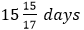and A and B together can complete same piece of work in 22 ½ days. B is 40% less efficient than A. Find in how many days A alone can complete the same work, if he is working 20% more efficiently?
(a) 20 days
(b) 28 days
(c) 32 days
(d) 30 days
(e) 25 days

Q5. Four persons started to do a work together. ‘A’ works only in starting two days after that B, C and D works alternately starting from B. Ratio of time taken by A, B, C and D if they work alone is 4 : 3 : 2 : 5. If the work is completed in 12 days then in how many days A and C can complete the work if they work together ?
(a) 6 days
(b) 12 days
(c) 10 days
(d) 8 days
(e) None of these

Q6. Abhishek and Abhinandan together can complete a work in 20 days. Abhinandan started to work alone and completes ⅔ work and remaining work is completed by Abhishek alone. They completed the work in total 39 days. Find in how many days Abhinandan alone can complete the work if he works for whole number of days?
(a) 45 days
(b) 36 days
(c) 30 days
(d) 24 days
(e) 60 days

Q7.  Veer takes 8 more days than Ayush to complete a work. Ankit is 20% more efficient than Ayush and takes two less days than Ayush takes. Find the number of days taken by all of them to complete twice this work together?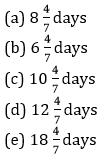Q8. A and B started the work alternatively starting with A. On last day, A completed the work by doing 12.5% of the whole work. Which of the following can be the possible value of time taken by B alone to do that work if A alone can do the whole work in 6 days.
(a) 15 days
(b) 8 days
(c) 10 days
(d) 6 days
(e) 12 days

Q9. A can do a piece of work in 48 days and B can do the same work in 64 days, while C take as half time as A and B take together. If they start working alternatively, starting by C, followed by B and then A respectively, then find in how many days work will be completed?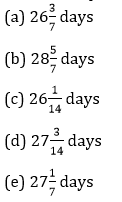Q10. P and Q together can complete a work in 24 days, while Q and R working together can complete the same job in 32 days. P and Q started the work and did it for 8 days, after that P left the work and R joined Q and after 12 more days, Q also left the work. Then, the remaining work was completed by R in 28 days. Find in how many days R will complete the work alone?
(a)  96 days
(b)  72 days
(c)  108 days
(d)  90 days
(e) 81 days

Q11.    Raman invested some amount in scheme ‘P’ which offer 20% C.I. p.a while some amount in scheme ‘Q’ which offers 8% S.I. p.a. After 2 year ratio of interest, earn from ‘P’ to ‘Q’ is 11 : 6.  Amount invested by Raman in scheme ‘P’ is what percent of the amount invested by him in scheme ‘Q’.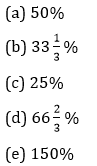Q12.  A man invested an amount at the rate of 20% p.a. on compound interest for two years. After two years he added 75% of the initial amount to the amount obtained and invested this amount at same rate of interest. If man got  a total of Rs. 3512 as interest after three years, find the amount received by man after three years, if he invested same amount on simple interest at rate of 15% p.a.?
(a) 5800 Rs.
(b) 5600 Rs.
(c) 5000 Rs.
(d) 5200 Rs.
(e) None of these

Q13. Ratio of CI earned only for 3rd year to CI earned only for 2nd year on a amount Rs. x at R% per annum is 13/10 and CI earned on Rs. (x + 5000) in 2 years at the same rate is Rs.13800.  Approximately after how many years will Rs x becomes Rs.25350 when put at compound interest at the same rate of interest?
(a) 6 years
(b) 2 years
(c) 4 years
(d) 3 years
(e) 7 years

Q14. Ritu invested her total saving in three different FD schemes A, B and C in the ratio of 5 : 4 : 6 on CI for two years at the rate of 10%, 15% and 20% respectively. If interest is calculated annually and interest from scheme B is Rs. 744 more than interest from scheme A then, find difference between interest received from scheme C and scheme B by Ritu?
(a) Rs. 4185
(b) Rs. 4175
(c) Rs. 3840
(d) Rs. 4580
(e) Rs. 3250

Q15.    Interest earn on an amount at 11% rate in simple interest for 4 years equal to the interest earn when same amount invested at some interest in a scheme with C.I. for 2 years. If Interest earn in C.I. for 3 years is 10920 then find out the initial amount invested.     (a) 9200
(b) 10500
(c) 15000
(d) 11000
(e) None of these

Practice More Questions of Quantitative Aptitude for Competitive Exams:

Solutions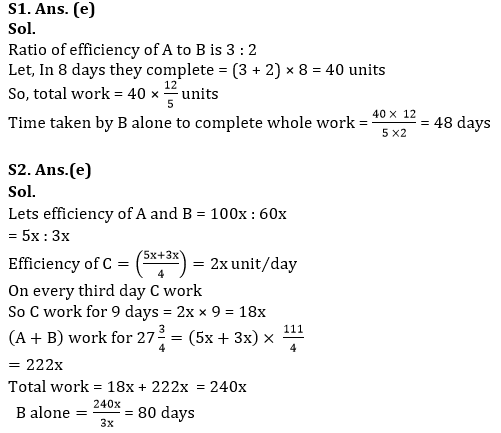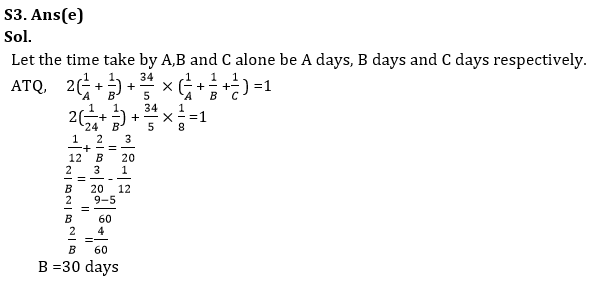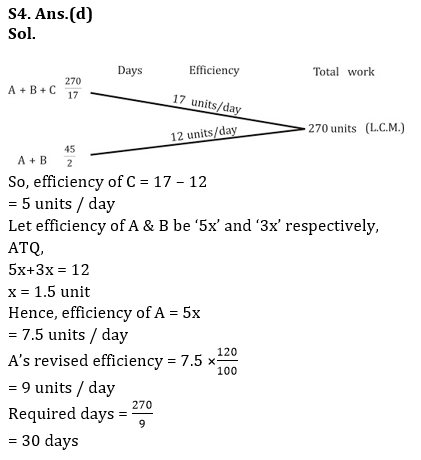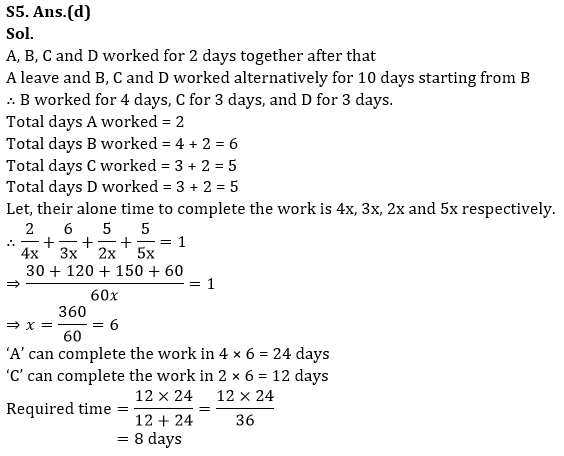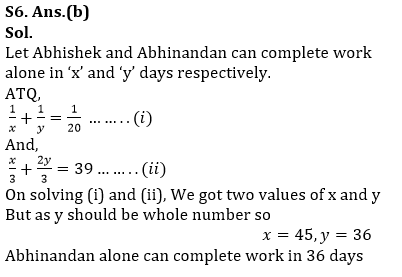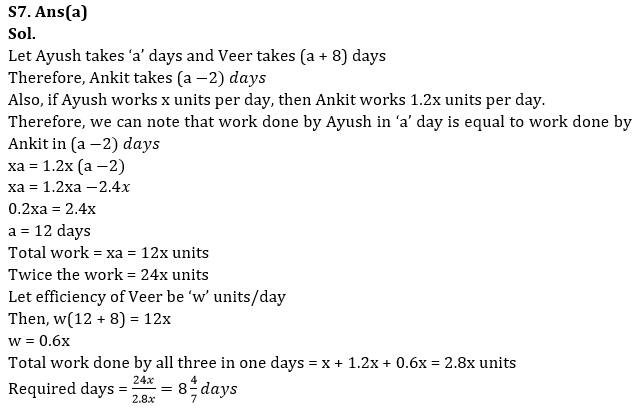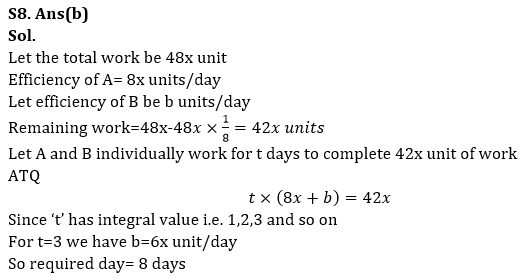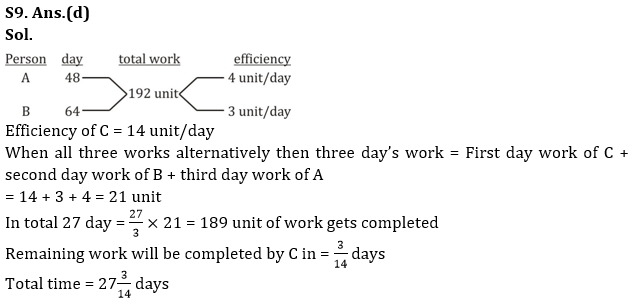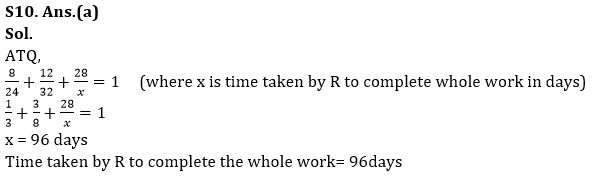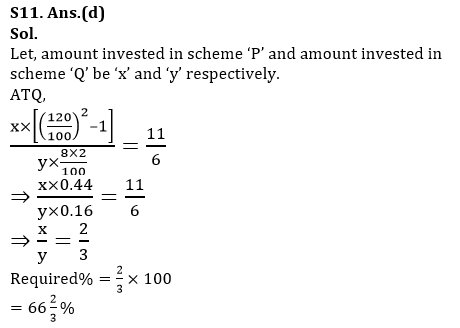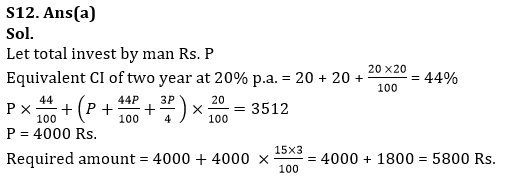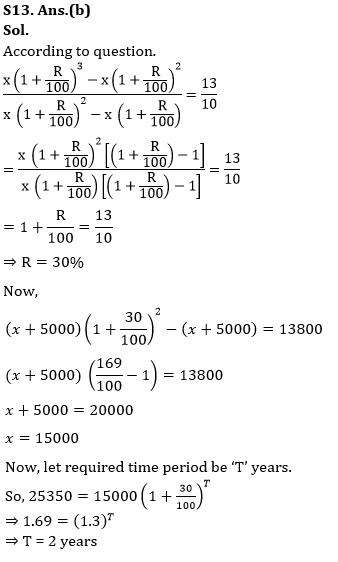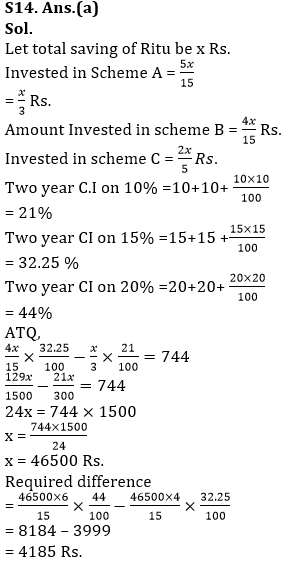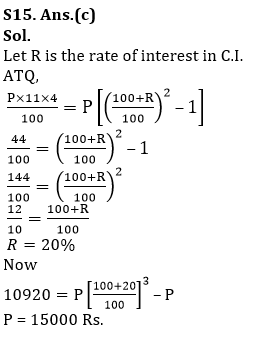×

Thank You, Your details have been submitted we will get back to you.Join India's largest learning destination

What You Will get ?

•Job Alerts
•Daily Quizzes
•Subject-Wise Quizzes
•Current Affairs
•Previous year question papers
•Doubt Solving session

ORJoin India's largest learning destination

What You Will get ?

•Job Alerts
•Daily Quizzes
•Subject-Wise Quizzes
•Current Affairs
•Previous year question papers
•Doubt Solving session

ORJoin India's largest learning destination

What You Will get ?

•Job Alerts
•Daily Quizzes
•Subject-Wise Quizzes
•Current Affairs
•Previous year question papers
•Doubt Solving session

Enter the email address associated with your account, and we'll email you an OTP to verify it's you.Join India's largest learning destination

What You Will get ?

•Job Alerts
•Daily Quizzes
•Subject-Wise Quizzes
•Current Affairs
•Previous year question papers
•Doubt Solving session

Enter OTP

Please enter the OTP sent to
/6

Did not recive OTP?

Resend in 60sJoin India's largest learning destination

What You Will get ?

•Job Alerts
•Daily Quizzes
•Subject-Wise Quizzes
•Current Affairs
•Previous year question papers
•Doubt Solving sessionJoin India's largest learning destination

What You Will get ?

•Job Alerts
•Daily Quizzes
•Subject-Wise Quizzes
•Current Affairs
•Previous year question papers
•Doubt Solving session

Almost there

+91Join India's largest learning destination

What You Will get ?

•Job Alerts
•Daily Quizzes
•Subject-Wise Quizzes
•Current Affairs
•Previous year question papers
•Doubt Solving session

Enter OTP

Please enter the OTP sent to Edit Number

Did not recive OTP?

Resend 60

By skipping this step you will not recieve any free content avalaible on adda247, also you will miss onto notification and job alerts

Are you sure you want to skip this step?

By skipping this step you will not recieve any free content avalaible on adda247, also you will miss onto notification and job alerts

Are you sure you want to skip this step?